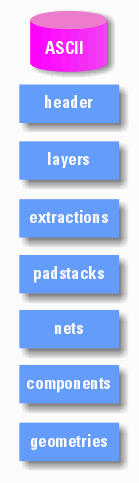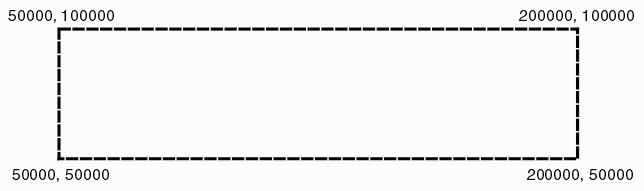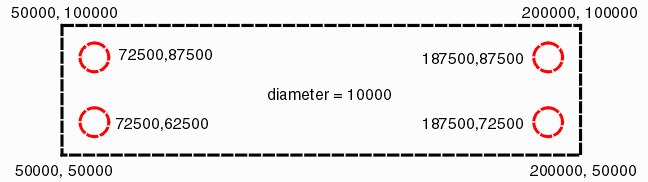# ASCII Output

NETEX-G,NETEX-ODB (and GBRUNion) can write out results as an ASCII file. The ASCII file can be as simple as just a list of polygons (along with header information) or it can contain component, pin, net, padstack and even wire elements.

The ASCII file has multiple sections as shown in the illustration at right:

header - contains units and resolution information

layers - layer stackup, thickness, material and electrical properties

extractions - if a net or window is to be extracted, it is specified here

nets - a table of nets either generated by NETEX or labeled in the input data

components - if extracted, component REF designator, location, pin list and attributes

geometries - list of geometries (sorted by net) with layer property. includes boundaries, leonov polygons (boundaries with holes), paths, vias and wires.The header describes ASCII version, whether there are any sections such as component libraries and the units/resolution of the geonmetry data.

```B_VERSION
2.00
HAS_COMP_LIB    Includes a component library section
E_ATTR
E_VERSION

B_UNITS         Units Section
UNITS INCH      Data Units
GRID 1000000    Data Resolution
E_UNITS
```

## Layers

This table contains a stackup position which is referred to by geometric entities such as boundaries and paths as well as padstacks and component placements; a layer type and a layer thickness. It is also possible for the user to include a material and associated eletrical properties.

An example of a layer table is shown below

```B_LAYERS
1   M1       METAL       0.000 GOLD     0xFF0000 0.000000  0.000000 0.000
2   D2       DIELECTRIC  0.000 TEFLON   0x00FF00 0.000000  0.000000 0.000
3   M3       METAL       0.000 GOLD     0xFF0000 0.000000  0.000000 0.000
4   D4       DIELECTRIC  0.000 TEFLON   0x00FF00 0.000000  0.000000 0.000
5   M5       METAL       0.000 GOLD     0xFF0000 0.000000  0.000000 0.000
6   D6       DIELECTRIC  0.000 TEFLON   0x00FF00 0.000000  0.000000 0.000
7   M7       METAL       0.000 GOLD     0xFF0000 0.000000  0.000000 0.000
E_LAYERS
```

Column Syntax

```Column 1 - layer stackup position
Column 2 - layer name (string - no spaces or special characters)
Column 3 - layer type (METAL | DIELECTRIC | WIREBOND | SOLDERMASK)
Column 4 - layer thickness
Column 5 - layer material e.g. copper, gold, FR4, teflon, air,
Column 6 - layer color ( RGB HEX 000000=black FFFFFF=white)
Column 7 - material conductivity
Column 8 - permittivity
Column 9 - permeability
```

A boundary on a dielectric layer should be interpreted as a via between two conductor layers -- not as a piece of dielectric. The NETEX-G output only consists of conductors.

## Silhouette

If any of the conductor layers are to be replaced with a "solid" sheet of metal, these layers would be listed here. This is often used for 3D modeling output where information about the internal layers is not needed but one wishes to keep them to preserve the board thickness. This section is optional.

```B_SILHOUETTE
1              indicates stackup pos 1 has been silhouetted
3              indicates stackup pos 1 has been silhouetted
E_SILHOUETTE
```

## Auxiliary Properties

This section points to "auxiliary" layers that are not part of the conductor stackup. There are a number of types including Solder Mask, Solder Paste and Silkscreen. This section is optional.

```B_AUX_PROPS
1   SM1   SOLDERMASKTOP 0.001370 Polyamide    0xFF8080FF 1e-014 3.3 1
2   SM2   SOLDERMASKBOT 0.001370 Polyamide    0xFF8080FF 1e-014 3.3 1
E_AUX_PROPS
```

>

## Shapes

Shapes is a new section introduced in November 2011. It was introduced in order to support more complete 3D models. This section will hold all sorts of layer related data that is not part of the conductor stackup.

Shapes could be used for a) board outline [profile] data, complex component outlines, complex padstack outlines, non-electical manufacturing layers such as soldermask, paste mask, route layers and such.

Therefore within the SHAPE section will be further subsections that describe the particular meaning or usage of the shape.

### Profile

This is a special shape that is used to define the profile of a printed circuit board. (it derives its name from the ODB++ data named profile.)

The board profile acts as a clipping boundary for any substrates and conductor layers defined as part of the board. Component outlines that leak out beyond the profile are not clipped since it is not unusual for components such as connectors to extend outside the boundary.

A profile can have "holes" in it which would normally represent mounting holes for the PCB or regions routed out of the PCB.

The source data for a profile can be a combination of profile (from ODB++) combined with drill and route layers. NETEX-G/ODB performs the required boolean operations and compensation for router tool width so that the profile that is defined in the ASCII output is always done using zero width polygons. [see programmer's notes on routes ...]

Example 1 Syntax

A simple rectangular board outline

```B_PROFILE         <-- begin the board profile

POLYGON_COUNT 1   <-- contains one polygon
VERTEX_COUNT 5    <-- polygons has 5 vertices
POLARITY P        <-- polygon is positive
B_XY              <-- begin XY coordinates
50000,50000       <-- X1,Y1
200000,50000      <-- X2,Y2
200000,100000     <-- X3,Y3
50000,100000      <-- X4,Y4
50000,50000       <-- X1,Y1  last coordinate back to first coordinate
END_XY            <--end coordinates
END_PROFILE       <--end board profile

```Example 2 Syntax

A simple rectangular board outline with a cutout

```B_PROFILE         <-- begin the board profile

POLYGON_COUNT 2   <-- contains one polygon
VERTEX_COUNT 5    <-- polygons has 5 vertices
POLARITY P        <-- polygon is positive
B_XY              <-- begin XY coordinates
50000,50000       <-- X1,Y1
200000,50000      <-- X2,Y2
200000,100000     <-- X3,Y3
50000,100000      <-- X4,Y4
50000,50000       <-- X1,Y1  last coordinate back to first coordinate
END_XY            <--end coordinates

VERTEX_COUNT 5    <-- polygons has 5 vertices
POLARITY N        <-- polygon is negative
B_XY              <-- begin XY coordinates
100000,60000       <-- X1,Y1
150000,60000      <-- X2,Y2
150000,90000     <-- X3,Y3
100000,90000      <-- X4,Y4
100000,60000       <-- X1,Y1  last coordinate back to first coordinate
END_XY            <--end coordinates

END_PROFILE       <--end board profile

```Example 3

A simple rectangular board outline with 4 round mounting holes

```B_PROFILE         <-- begin the board profile

POLYGON_COUNT 5   <-- contains one polygon
VERTEX_COUNT 5    <-- polygons has 5 vertices
POLARITY P        <-- polygon is positive
B_XY              <-- begin XY coordinates
50000,50000       <-- X1,Y1
200000,50000      <-- X2,Y2
200000,100000     <-- X3,Y3
50000,100000      <-- X4,Y4
50000,50000       <-- X1,Y1  last coordinate back to first coordinate
END_XY            <--end coordinates

CIRCLE            <-- entity is a circle
POLARITY N        <-- entity is negative
B_XY              <-- begin XY coordinates
72500,62500,10000 <-- XCENTER,YCENTER,DIA
END_XY            <--end coordinates

CIRCLE            <-- entity is a circle
POLARITY N        <-- entity is negative
B_XY              <-- begin XY coordinates
72500,62500,10000 <-- XCENTER,YCENTER,DIA
END_XY            <--end coordinates

CIRCLE            <-- entity is a circle
POLARITY N        <-- entity is negative
B_XY              <-- begin XY coordinates
72500,62500,10000 <-- XCENTER,YCENTER,DIA
END_XY            <--end coordinates

CIRCLE            <-- entity is a circle
POLARITY N        <-- entity is negative
B_XY              <-- begin XY coordinates
72500,62500,10000 <-- XCENTER,YCENTER,DIA
END_XY            <--end coordinates

END_PROFILE       <--end board profile

```## Extractions

The extraction section is optional. If a user has specified a window to extract or a set of nets to extract the definition would be located in this section.

For extractions BYNAME (i.e. where a net or nets has been specified) an expansion distance may be specified also. If this expansion distance is specifed then the extraction program will "grab" any nearby conductors i.e. first the conductors specified by NAME are extracted and then any conductors close enough to influence the selected nets are extracted. If the expansion directive is missing or set to zero, then no adjacent conductors to the specified nets are extracted.

Currently this extraction section is only used by ASC23DI converter to the 3DI format.

```B_EXTRACTIONS

B_EXPANSION
4.000000
E_EXPANSION

B_EXTRACT BYNAME  "E:\vid\vid_nets.3di" PROXIMITY
VID*
E_EXTRACT

E_EXTRACTIONS
```

Padstacks are generated when the input to NETEX-G is ODB++. Vias in the geometry section will refer back to a padstack as will component pins.

```
1 pin_def_1           <-- index, stack name
1  C 1.5  1.5  1.0 P  <-- stackup, shape, xdim,ydim, drill dia, layer polarity (P|N)
7  C 1.5  1.5  1.0 P  <-- stackup, shape, xdim,ydim, drill dia

2 pin_def_2         <-- start of next padstack
1  R 1.5  1.3 0 P
```

## Net Table

A net table is provided so that other entities such as vias and component pins can point back to an entry in the table. There are two columns to this table - the index (a positive integer) followed by the character string representing the full net name.

Net names may be generated automatically by NETEX-G if no label was found either from the ODB++ file netlist or from an external source such as IPC-D-356. NETEX-G creates net names using the syntax Net1, Net2, Net3 ... ODB++ often uses a label \$\$NONE\$\$ indicating that a particular conductor is not labeled and one should not assume that all conductors that share the name \$\$NONE\$\$ are physically or electrically connected.

```B_NET_TABLE
1    Net1
2    GND
3    KEY3
4    KEY2
5    KEY1
6    KEY0
7    POS3_3V
8    Net8
9    Net9
10   Net10
11   Net11
12   Net12
13   Net13
14   Net14
15   Net15
16   NetLED1_2
17   NetLED2_2
18   NetLED3_2
19   NetLED4_2
20   AIN0
21   AIN1
22   AIN2
23   LED3
24   LED2
25   Net25
26   Net26
27   Net27
.
.
.
B_NET_TABLE
```

## Components

Beginning in version 1.49 the ASCII output of NETEX-G can contain a component section - in particular when the input to NETEX-G is ODB++ and the ODB++ file has a netlist section.

A component entry is shown below. Notice that we do not take a library/reference point of view -- instead each placed component has its own complete entry.

```B_COMP_REF                                   <-- beginning of a component placement
U8  152.956 158.877 1                        <-- ref_des x,y stackup
COMP_NAME SOIC8                              <-- name of the component from ODB
COMP_EXTENT 149.206 156.722 156.706 161.032  <-- comp extent box (absolute)
B_COMP_ATTR                                  <-- start of any attributes found in ODB
comp_mount_type 1                            <-- taken directly from ODB++
comp_height 0.0000                           <-- taken directly from ODB++
E_COMP_ATTR                                  <-- end of component attributes
B_COMP_PIN                                   <-- start listing of component pins ...
1 155.9562  156.972 1 4 180 M0    7         <-- pin_label x,y stackup padstack rotation mirror net
2 155.9562  158.242 1 4 180 M0  163         <-- pin_label x,y stackup padstack rotation mirror net
3 155.9562  159.512 1 5 180 M0  185
4 155.9562  160.782 1 6 180 M0    2
5 149.9562  160.782 1 6 180 M0   66
6 149.9562  159.512 1 5 180 M0   85
7 149.9562  158.242 1 4 180 M0  177
8 149.9562  156.972 1 4 180 M0    7
E_COMP_PIN                                   <-- end of pin listing
E_COMP_REF                                   <-- end of component placement definition

Proposed Change to COMP_ROTATION (July 20, 2010)

COMP_EXTENT DX DY INSx INSy ROT MFLAG

where

DX DY      are the width and height for the untransformed comp outline
INSx INSy  define the insertion center of the outline
ROT        defins the rotation (CCW from X axis)
MFLGAG     defines mirror (doubtful if needed)

```

## The Geometry Section

This was the "original" data output to Atwork's ASCII file and hence has no bracketed keyword. However you can recognize it by the string LIBRARY in the first line. Because of its GDSII origins, some of the data in this section maps the architecture of GDSII stream.

Library Line

The first line in the geometry section is the library line (from its GDSII origins). This contains:

the keyword LIBRARY
the "name" of the library
the database units
the database grid

All coordinate data in this section is to be divided by the grid value and then intepreted in the declared units. Since GDSII's native resolution is 10-9 meter (1 nanometer) valid combinations of units and grid cannot be finer than 1 nm. i.e. you can specify microns with a grid of 1000 but you cannot legally specify microns with a grid of 10,000.

`LIBRARY PBGA unit:MM grid:1000000`

In the example above, the libray name is PBGA, and the coordinate data is in millimeters. Divide each ASCII coordinate by 1000000 to compute the value in mm. An ascii value of 5000 = 0.005 mm

Sorted by NET

Geometries present in this section are sorted by net. All geometries following a NET line belong to that net. The minimum amount of information on the net line is just the name of the NET

`NET Net1`

Nodes

The net name can be followed by a series of "nodes" often called "pins" in PCB applications. This node information is derived either from IPC-D-356 test point files, AIF file or from a ODB++ file's netlist section.

` NET Net1 BF2 1164800 937500 1`

The syntax for a node is as follows:

node name (a string with no spaces)
node X coord
node Y coord
stackup position (i.e. the layer that the node is defined on)

In the example above, the node (or pin) on Net1 is called BF2 and it is located at 1164800,937500 and it occurs on stackup (layer) 1.

It is possible and generally likely to have many nodes on a NET line especially if it is a power or ground net.

### Boundary

A very common geometry entity is the boundary. It consists of a series of vertex/segments (no arcs) that define a closed region. The last vertex is assumed to connect or close to the first vertex. A boundary should not self intersect though in the limiting case it may self touch.

```
BOUNDARY 1       <-- boundary keyword followded by stackup (layer) position
1173867 9384616  <-- first vertex
1165991 9375977  <-- next vertex
1160681 9362270  <-- next vertex
1160681 9347570  <-- next vertex
1165991 9333863  <-- next vertex; segment automatically conntects to first vertex
ENDEL            <-- ends the boundary definition

```

### LEONOV Polygon

If you look at the boundary definition you can see that there is no provision for holes or islands/voids within the boundary. However many PCB databases support such a description. Therefore there is a second form of boundary that does support holes. We call these Leonov polygons in honor of the Russian mathematician that wrote a seminal paper on the topic.

Leonov polygons are grouped with a container boundary coming first in the list followed by one or more children boundaries. The children are "subtractive" - their area is subtracted from the container polygon. In order to identify such polygons in the ASCII output a special header is used: BGNLEONOV to start the group and ENDLEONOV to close the group.

```BGNLEONOV        <-- beginning of the group.

BOUNDARY 1       <-- the container boundary.
1173867 9384616
1165991 9375977
1160681 9362270
1160681 9347570
1165991 9333863
ENDEL

BOUNDARY 1       <--  a child boundary.
1173867 9384616
1165991 9375977
1160681 9362270
1160681 9347570
1165991 9333863
ENDEL

BOUNDARY 1       <-- child boundary.
1273867 9484616
1265991 9475977
1260681 9462270
1260681 9447570
1265991 9433863
ENDEL

ENDLEONOV        <-- end of the group.

```

An example ASCII file containing a number of regular boundaries and Leonov polygons can be found on this page describing the correct interpretation of Leonov polygons.

### PATH

The ASCII format now supports paths. These are lost during the initial stages of conversion due to Boolean operations but are then "recovered" from the resulting boundary data; occasionally the endpoint of the path may not match the original endpoint from the Gerber/ODB data when terminating on a pad. Because the source data for paths Gerber/ODB++ one should assume that the path is created by a circular draw.

```
PATH 7 0 0 1150000     <-- keyword, stackup, datatype, endcap, width
-1026883648 1027675000 <-- x,y coordinate
-1026883648 1026525000 <-- x,y coordinate
ENDEL                  <-- end of element definition

```
Notes

datatype: (GDSII parameter only, integer 0-4096 not used ...)

endcap: 0=flush, 1 = round 2=1/2 ext square

Vias

There are two approaches to handling vias: by default, each via is decomposed into its crossection (a circular shaped polygon) on the layer that it passes through; optionally, one can also get a "symbolic insertion of a via in which the start and end layer are specified along with the via's diameter.

The via syntax is described below:

```
VIA 1 5 10000     <-- via from stackup 1 through stackup 5
<-- diameter = 10000 see library for units
5685000 4245000   <-- center of via
ENDEL             <-- end of via

VIA 1 5 10000 43  <-- a fourth argument may be present
it would be the padstack. In that case
ignore the via diameter and use the
5425000 3985000
ENDEL

```

### Wirebonds

Starting with Version 1.40 of NETEX-G the Wirebond data has been greatly expanded to support a full 3D description and is now described on its own page.

Wirebonds are not normally converted to boundaries unless the user sets them as METAL layers instead of WIREBOND layers in the stackup definition. Instead, NETEX-G outputs a special WIRE entity that includes the layer, diameter and starting/ending coordinates of the wire.

```WIRE 3 25400 W3
-3937800 1580000
-5826180 2441410
ENDEL
```

Syntax

```column 1 - WIRE
column 2 - stackup layer
column 3 - wire diameter
column 4 - layer name
```

Wire Coordinates

Wire coordinates are automatically sorted by NETEX-G. The first pair define "inner" end of the wire with the assumption made that these connect to the die side. The second pair define the "outer" end of the wire with the assumption that they drop down to the metal below.